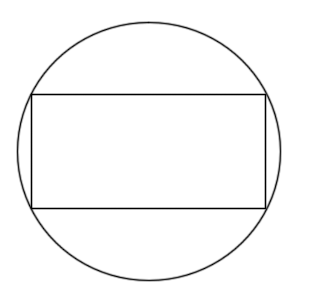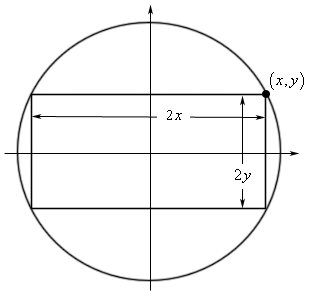Paul's Online Notes
Home / Calculus I / Applications of Derivatives / More Optimization Problems
Show Mobile Notice Show All Notes Hide All Notes
Mobile Notice
You appear to be on a device with a "narrow" screen width (i.e. you are probably on a mobile phone). Due to the nature of the mathematics on this site it is best views in landscape mode. If your device is not in landscape mode many of the equations will run off the side of your device (should be able to scroll to see them) and some of the menu items will be cut off due to the narrow screen width.

### Section 4.9 : More Optimization

2. Determine the area of the largest rectangle that can be inscribed in a circle of radius 1.Show All Steps Hide All Steps

Start Solution

Let’s start with a quick sketch of the circle and rectangle. Also, in order to make the work a little easier we went ahead and assumed that the circle was centered at the origin of the standard $$xy$$-coordinate system.

We’ve also defined a point $$\left( {x,y} \right)$$ in the first quadrant. This is the point that we will be attempting to find when we get into the problems. If we know the coordinates of this point then the rectangle defined by the point, as shown in the figure, will be the one with the largest area.Show Step 2

Next, we need to set up the constraint and equation that we are being asked to optimize.

Given our graph above we can easily determine the equation of the circle. This will also be the constraint of the problem because the corners of the rectangle must be on the circle.

${x^2} + {y^2} = 1$

Also note that from the figure or equation we can clearly see that $$- 1 \le x \le 1$$ and $$- 1 \le y \le 1$$. One or both of these limits will be useful later on in the problem.

We are being asked to maximize the amount of the rectangle and using the definitions we see in the figure above the area is,

$A = \left( {2x} \right)\left( {2y} \right) = 4xy$ Show Step 3

We can solve the constraint for $$x$$ or $$y$$. Either will lead to essentially the same work so we’ll solve for $$x$$.

$x = \pm \sqrt {1 - {y^2}}$

Because we’ve defined the point on the circle to be in the 1st quadrant we will use the “+” portion of this. Plugging this into the area function gives,

$A\left( y \right) = 4y\sqrt {1 - {y^2}}$ Show Step 4

Finding the critical point(s) for this shouldn’t be too difficult at this point. Here is the derivative.

$A'\left( y \right) = 4\sqrt {1 - {y^2}} - \frac{{4{y^2}}}{{\sqrt {1 - {y^2}} }} = \frac{{4 - 8{y^2}}}{{\sqrt {1 - {y^2}} }}$

From this it looks like, from the numerator, we get the critical points,

$y = \pm \sqrt {{\frac{1}{2}}} = \pm \frac{1}{\sqrt{2}} = \pm 0.7071$

From the denominator we get the critical points : $$y = \pm 1$$ and yes these are critical points because the function will exist at these points.

Before proceeding to the next step let’s notice that because our point is in the first quadrant we know that $$y$$ must be positive. This fact along with the limits on $$y$$ we discussed in Step 2 tells us that we must have : $$0 \le y \le 1$$.

This in turn tells us that the only two critical points that we need to worry about are,

$y = \frac{1}{\sqrt{2}} = 0.7071\hspace{0.5in}y = 1$ Show Step 5

Because we’ve got a range for possible critical points all we need to do to determine the maximum area is plug the end points and critical points into the area.

$A\left( 0 \right)\hspace{0.5in}A\left( \frac{1}{\sqrt{2}} \right) = 2\hspace{0.5in}A\left( 1 \right) = 0$ Show Step 6

So, the area of the largest rectangle that can be inscribed in the circle is : 2.